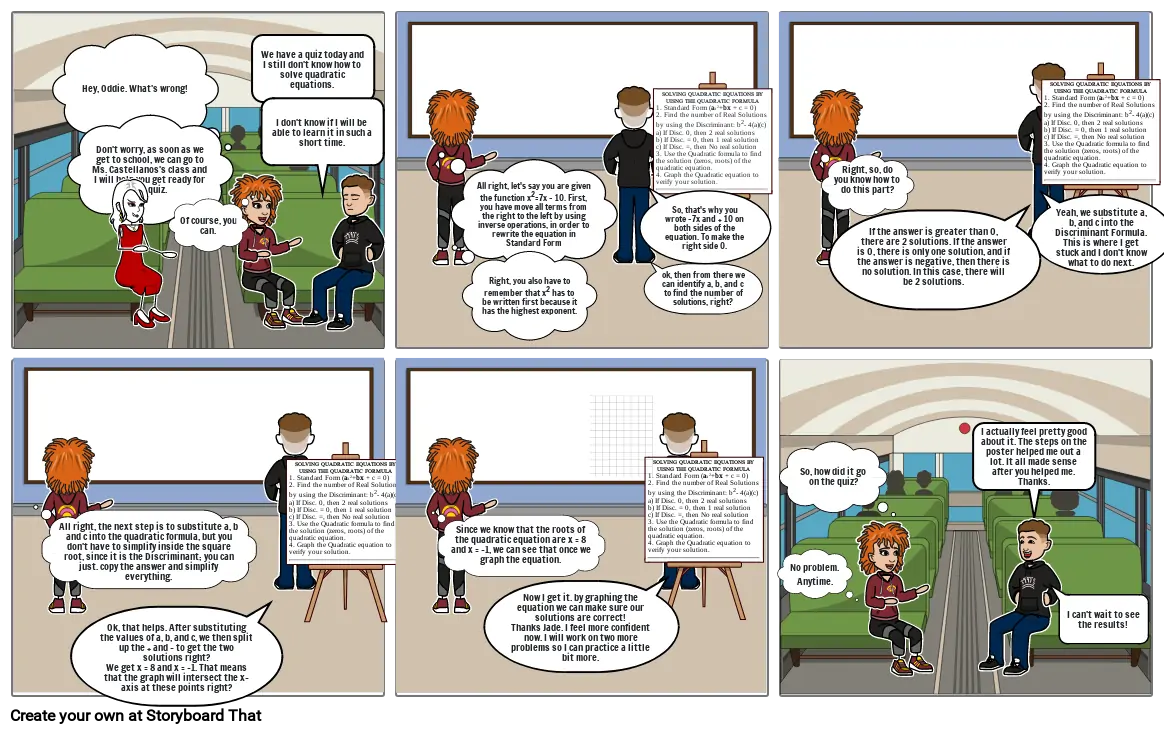Create a Storyboard#### Storyboard Text

• Hey, Oddie. What's wrong!
• Don't worry, as soon as we get to school, we can go to Ms. Castellanos's class and I will help you get ready for the quiz.
• Of course, you can.
• We have a quiz today and I still don't know how to solve quadratic equations.
• I don't know if I will be able to learn it in such a short time.
• All right, let's say you are given the function x2=7x - 10. First, you have move all terms from the right to the left by using inverse operations, in order to rewrite the equation in Standard Form
• Right, you also have to remember that x2 has to be written first because it has the highest exponent.
• So, that's why you wrote -7x and + 10 on both sides of the equation. To make the right side 0.
• ok, then from there we can identify a, b, and c to find the number of solutions, right?
• SOLVING QUADRATIC EQUATIONS BY UISNG THE QUADRATIC FORMULA1. Standard Form (ax2+bx + c = 0)2. Find the number of Real Solutions by using the Discriminant: b2- 4(a)(c) a) If Disc. 0, then 2 real solutions b) If Disc. = 0, then 1 real solution c) If Disc. =, then No real solution3. Use the Quadratic formula to find the solution (zeros, roots) of the quadratic equation. 4. Graph the Quadratic equation to verify your solution.
• Right, so, do you know how to do this part?
• If the answer is greater than 0, there are 2 solutions. If the answer is 0, there is only one solution, and if the answer is negative, then there is no solution. In this case, there will be 2 solutions.
• Yeah, we substitute a, b, and c into the Discriminant Formula. This is where I get stuck and I don't know what to do next.
• SOLVING QUADRATIC EQUATIONS BY UISNG THE QUADRATIC FORMULA1. Standard Form (ax2+bx + c = 0)2. Find the number of Real Solutions by using the Discriminant: b2- 4(a)(c) a) If Disc. 0, then 2 real solutions b) If Disc. = 0, then 1 real solution c) If Disc. =, then No real solution3. Use the Quadratic formula to find the solution (zeros, roots) of the quadratic equation. 4. Graph the Quadratic equation to verify your solution.
• All right, the next step is to substitute a, b and c into the quadratic formula, but you don't have to simplify inside the square root, since it is the Discriminant; you can just. copy the answer and simplify everything.
• Ok, that helps. After substituting the values of a, b, and c, we then split up the + and - to get the two solutions right?We get x = 8 and x = -1. That means that the graph will intersect the x-axis at these points right?
• SOLVING QUADRATIC EQUATIONS BY UISNG THE QUADRATIC FORMULA1. Standard Form (ax2+bx + c = 0)2. Find the number of Real Solutions by using the Discriminant: b2- 4(a)(c) a) If Disc. 0, then 2 real solutions b) If Disc. = 0, then 1 real solution c) If Disc. =, then No real solution3. Use the Quadratic formula to find the solution (zeros, roots) of the quadratic equation. 4. Graph the Quadratic equation to verify your solution.
• Since we know that the roots of the quadratic equation are x = 8 and x = -1, we can see that once we graph the equation.
• Now I get it. by graphing the equation we can make sure our solutions are correct!Thanks Jade. I feel more confident now. I will work on two more problems so I can practice a little bit more.
• SOLVING QUADRATIC EQUATIONS BY UISNG THE QUADRATIC FORMULA1. Standard Form (ax2+bx + c = 0)2. Find the number of Real Solutions by using the Discriminant: b2- 4(a)(c) a) If Disc. 0, then 2 real solutions b) If Disc. = 0, then 1 real solution c) If Disc. =, then No real solution3. Use the Quadratic formula to find the solution (zeros, roots) of the quadratic equation. 4. Graph the Quadratic equation to verify your solution.
• No problem. Anytime.
• So, how did it go on the quiz?
• I actually feel pretty good about it. The steps on the poster helped me out a lot. It all made sense after you helped me. Thanks.
• I can't wait to see the results!
Over 30 Million Storyboards Created